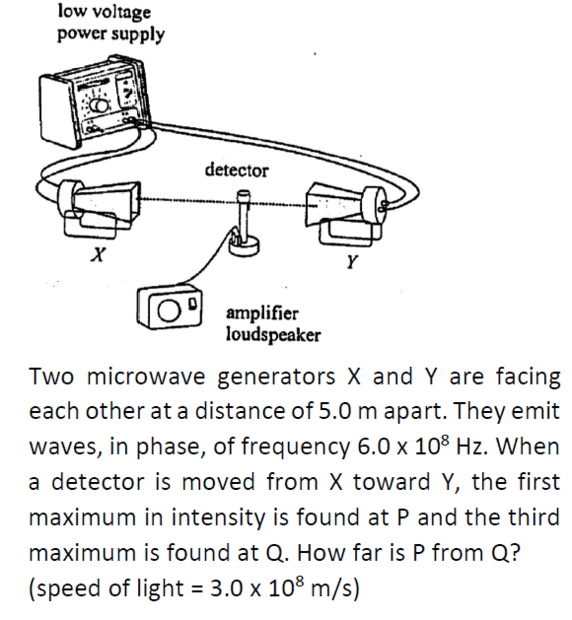# Distance between the first and third maximum intensity detected

• songoku

#### songoku

Homework Statement
Relevant Equations
Not sureMy answer is 0.5 m (same as wavelength) but answer key is 1 m.

I thought the maximum intensity occurs at antinode and distance between adjacent antinodes is half of wavelength so the distance between first and third antinode is one wavelength.

Where is my mistake? Thanks

Your reasoning seems good to me. The distance between consecutive maxima should be half a wave length so the distance between the first and the third should be a full wave length.

•songoku
Thnk you very much Orodruin

I think that the answer key answer was erroneously arrived at by starting at the criterion for constructive interference that the path length difference is an integer number of wavelengths. Then one could argue that If one starts at the midpoint and moves to the left by half a wavelength, the path length difference will be a full wavelength and two full wavelengths (1.0 m) if one skips the maximum in-between. However, this argument is flawed because the waves are traveling in opposite directions and a standing wave is set up. This can be confirmed mathematically by writing formal expressions for each wave and adding them.

•songoku
I don’t think it matters much exactly how the answer key got it wrong as much as the correct argument:

Letting the distance between the sources be ##L## and the distance of a point on the line between the sources to the left source ##x##, constructive interference occurs when the distances to the sources equal an integer ##n## wavelengths ##\lambda##, i.e., when
$$(L-x)-x = n\lambda \quad \Leftrightarrow \quad x = \frac L2 - \frac{n\lambda}{2}.$$
Consequently, the difference in ##x## for consecutive ##n## is ##\lambda/2##.

•songoku